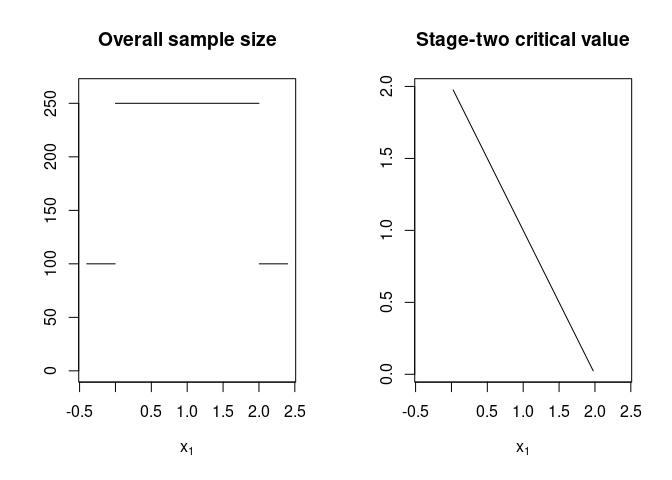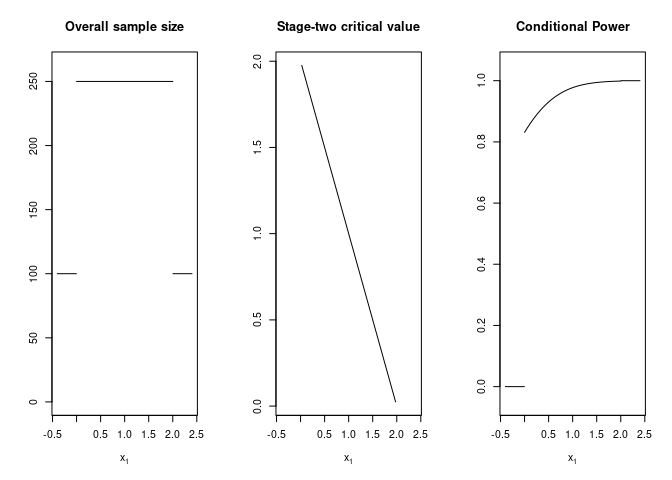# Conditional Scores and Constraints

library(adoptr)

There are two fundamental ways of scoring a two-stage design: First, one may assess the performance before observing any data, i.e., at the planning stage. Classical examples for such scores would be power, type-one-error rate, or expected sample size. There is, however, a second perspective. After observing the stage-one outcome, one might be inclined to consider conditional properties of a design. The most prominent example being conditional power (probability to reject the null under the alternative given stage-one outcome). We consider the following example design

design <- TwoStageDesign(
n1        = 100,
c1f       = .0,
c1e       = 2.0,
n2_pivots = rep(150, 5),
c2_pivots = sapply(1 + adoptr:::GaussLegendreRule(5)\$nodes, function(x) -x + 2)
)
plot(design)In adoptr, scores are instances of their respective score class. The most important ones are: ConditionalScore, UnconditionalScore, and IntegralScore. An object of class ConditionalScore can evaluate a design for a particular stage-one outcome. A ConditionalScore is a function $$s(\mathcal{D}, x_1)$$ evaluating a design $$\mathcal{D}$$ at a stage-one outcome $$X_1 = x_1$$. Some conditional scores might depend on the data distribution (conditional power) others do not (conditional sample size). Conditional score evaluation is completely vectorized:

uniform_prior <- ContinuousPrior(
function(x) numeric(length(x)) + 1/.2,
support = c(.3, .5)
)

cp  <- ConditionalPower(Normal(), uniform_prior)
css <- ConditionalSampleSize()

x1  <- c(0, .5, 1)
evaluate(cp, design, x1)
#>  0.8312538 0.9303985 0.9772962
evaluate(css, design, x1)
#>  250 250 250

Conditional scores can also be plotted directly for a given design by including them in the plot() call.

plot(design, "Conditional Power" = cp)Any conditional score can be integrated with respect to a prior and data distribution to obtain an unconditional score. Note that for conditional scores which depend on a specification of these distributions (e.g., conditional power) these arguments must be consistent! The resulting score is of class IntegralScore, a specific subclass of UnconditionalScore and evaluates designs independent of a particular $$x_1$$, i.e., unconditionally

ep <- expected(cp, Normal(), uniform_prior)
evaluate(ep, design)
#>  0.989392

Power at a point alternative can be obtained by forming the expected value with respect to a point prior. For convenience, we include a constructor for power directly, e.g., both variants are equivalent and give power at $$0.4$$.

power1 <- expected(
ConditionalPower(Normal(), PointMassPrior(.4, 1.0)),
Normal(), PointMassPrior(.4, 1.0)
)
power2 <- Power(Normal(), PointMassPrior(.4, 1.0))

evaluate(power1, design)
#>  0.9967857
evaluate(power2, design)
#>  0.9967857

Similarly, ExpectedSampleSize is a shorthand constructor for expected conditional sample size, i.e., the overall expected sample size:

ess1 <- expected(ConditionalSampleSize(), Normal(), uniform_prior)
ess2 <- ExpectedSampleSize(Normal(), uniform_prior)

evaluate(ess1, design)
#>  134.9135
evaluate(ess2, design)
#>  134.9135

## Conditional Constraints

The same syntax for constraint specification as for unconditional constraints (power etc.) can be used for conditional scores (e.g. conditional power) as well. Currently, these constraints apply to the continuation area only. E.g.,

cp >= 0.7
#> -Pr[x2>=c2(x1)|x1] (x1) <= -0.7 for x1 in [c1f,c1e]

imposes a constraint on the minimal conditional power upon continuation. Inter-score comparisons are also supported, e.g.

cp >= ConditionalPower(Normal(), PointMassPrior(0, 1))
#> Pr[x2>=c2(x1)|x1]  - Pr[x2>=c2(x1)|x1] (x1) <= 0 for x1 in [c1f,c1e]

Would enforce that conditional power is always larger than conditional error.